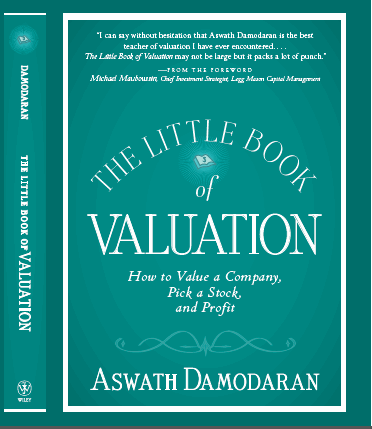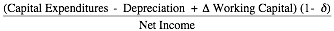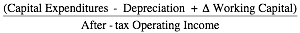#The Little Book of Valuation

### Cash Flows

Cash flows can be estimated to either just equity investors (cash flows to equity) or to all suppliers of capital (cash flows to the firm). In this section, we will begin with the strictest measure of cash flow to equity, i.e. the dividends received by investors, and then progressively move to more expansive measures of cash flows, which generally require more information.

#### Dividends

When an investor buys stock, he generally expects to get two types of cash flows - dividends during the holding period and an expected price at the end of the holding period. Since this expected price is itself determined by future dividends, the value of a stock is the present value of just expected dividends. If we accept this premise, the only cash flow to equity that we should be considering in valuation is the dividend paid, and estimating that dividend for the last period should be a simple exercise. Since many firms do not pay dividends, this number can be zero, but it should never be negative.

#### Augmented Dividends

One of the limitations of focusing on dividends is that many companies, especially in the United States but increasingly around the world, have shifted from dividends to stock buybacks as their mechanism for returning cash to stockholders. While only those stockholders who sell their stock back receive cash, it still represents cash returned to equity investors. In 2007, for instance, firms in the United States returned twice as much cash in the form of stock buybacks than they did in dividends, and focusing only on dividends will result in the under valuation of equity. One simple way of adjusting for this is to augment the dividend with stock buybacks and look at the cumulative cash returned to stockholders.

Augmented Dividends = Dividends + Stock Buybacks

One problem, though, is that unlike dividends that are smoothed out over time, stock buybacks can spike in some years and be followed by years of inaction. We therefore will have to normalize buybacks by using average buybacks over a period of time (say, 5 years) to arrive at more reasonable annualized numbers.

#### Potential Dividends (Free Cash flow to Equity)

With both dividends and augmented dividends, we are trusting managers at publicly traded firms to return to pay out to stockholders any excess cash left over after meeting operating and reinvestment needs. However, we do know that managers do not always follow this practice, as evidenced by the large cash balances that you see at most publicly traded firms. To estimate what managers could have returned to equity investors, we develop a measure of potential dividends that we term the free cash flow to equity. Intuitively, the free cash flow to equity measures the cash left over after taxes, reinvestment needs and debt cash flows have been met. It is measured as follows:

FCFE = Net Income – Reinvestment Needs – Debt Cash flows

= Net Income + (Capital Expenditures - Depreciation + Change in non-cash working Capital – Principal) -  (Repayments + New Debt Issues)

Consider the equation in pieces. We begin with net income, since that is the earnings generated for equity investors; it is after interest expenses and taxes. We compute what the firm has to reinvest in two parts:

1. Reinvestment in long-lived assets is measured as the difference between capital expenditures (the amount invested in long lived assets during the period) and depreciation (the accounting expense generated by capital expenditures in prior periods). We net the latter because it is not a cash expense and hence can be added back to net income.
2. Reinvestment in short-lived assets is measured by the change in non-cash working capital. In effect, increases in inventory and accounts receivable represent cash tied up in assets that do not generate returns – wasting assets. The reason we done consider cash in the computation is because we assume that companies with large cash balances generally invest them in low-risk, marketable securities like commercial paper and treasury bills; these investments earn a low but a fair rate of return and are therefore not wasting assets. To the extent that they are offset by the use of supplier credit and accounts payable, the effect on cash flows can be muted. The overall change in non-cash working capital therefore is investment in short-term assets.

Reinvestment reduces cash flow to equity investors, but it provides a payoff in terms of future growth. We will come back and consider whether the net effect is positive or negative after we consider how best to estimate growth. The final input into the process are the negative cash flows associated with the repayment of old debt and the positive cash flows to equity investors from raising new debt. If old debt is replaced with new debt of exactly the same magnitude, this term will be zero, but it will generate positive (negative) cash flows when debt issues exceed (are less than) debt repayments.

Focusing on just debt cash flows allows us to zero in on a way to simplify this computation. In the special case where the capital expenditures and the working capital are expected to be financed at a fixed debt ratio d, and principal repayments are made from new debt issues, the FCFE is measured as follows:

FCFE = Net Income + (1-d) (Capital Expenditures - Depreciation) + (1-d) D Working        Capital

In effect, we are assuming that a firm with a 30% debt ratio that is growing through reinvestment will choose to fund 30% of its reinvestment needs with new debt and replace old debt that comes due with new debt.

There is one more way in which we can present the free cash flow to equity. If we define the portion of the net income that equity investors reinvest back into the firm as the equity reinvestment rate, we can state the FCFE as a function of this rate.

Equity Reinvestment Rate

=FCFE = Net Income (1 – Equity Reinvestment Rate)

A final note on the contrast between the first two measures of cash flows to equity (dividends and augmented dividends) and this measure. Unlike those measures, which can never be less than zero, the free cash flow to equity can be negative for a number of reasons. The first is that the net income could be negative, a not uncommon phenomenon even for mature firms. The second is that reinvestment needs can overwhelm net income, which is often the case for growth companies, especially early in the life cycle.  The third is that large debt repayments coming due that have to funded with equity cash flows can cause negative FCFE; highly levered firms that are trying to bring their debt ratios down can go through years of negative FCFE. The fourth is that the quirks of the reinvestment process, where firms invest large amounts in long-lived and short-lived assets in some years and nothing in others, can cause the FCFE to be negative in the big reinvestment years and positive in others. As with buybacks, we have to consider normalizing reinvestment numbers across time when estimating cash flows to equity. If the FCFE is negative, it is indicative of the firm needing to raise fresh equity.

#### Cash Flow to the Firm

The cash flow to the firm that we would like to estimate should be both after taxes and after all reinvestment needs have been met. Since a firm raises capital from debt and equity investors, the cash flow to the firm should be before interest and principal payments on debt. The cash flow to the firm can be measured in two ways. One is to add up the cash flows to all of the different claim holders in the firm. Thus, the cash flows to equity investors (estimated using one of the three measures described in this section) are added to the cash flows to debt holders (interest and net debt payments) to arrive at the cash flow. The other approach is to start with operating earnings and to estimate the cash flows to the firm prior to debt payments but after reinvestment needs have been met:

Free Cash flow to firm (FCFF) = After-tax Operating Income - Reinvestment

= After-tax Operating Income– (Capital Expenditures –Depreciation + Change in non-cash Working Capital)

It is easiest to understand FCFF by contrasting it with FCFE. First, we begin with after-tax operating income instead of net income; the former is before interest expenses whereas the latter is after interest expenses. Second, we adjust the operating income for taxes, computed as if you were taxed on the entire income, whereas net income is already an after-tax number. Third, while we subtract out reinvestment, just as we did to arrive at free cash flows to equity, we do not net out the effect of debt cash flows, since we are now looking at cash flows to all capital and not just to equity.

Another way of presenting the same equation is to cumulate the net capital expenditures and working capital change into one number, and state it as a percentage of the after-tax operating income. This ratio of reinvestment to after-tax operating income is called the reinvestment rate, and the free cash flow to the firm can be written as:

Reinvestment Rate =Free Cash Flow to the Firm = EBIT (1-t) (1 – Reinvestment Rate)

Note that the reinvestment rate can exceed 100%, if the firm has substantial reinvestment needs. The reinvestment rate can also be less than zero, for firms that are divesting assets and shrinking capital.

A few final thoughts about free cash flow to the firm are worth noting before we move on to discount rates. First, the free cash flow to the firm can be negative, just as the FCFE can, but debt cash flows can no longer be the culprit; even highly levered firms that are paying down debt will report positive FCFF while also registering negative FCFE. If the FCFF is negative, the firm will be raising fresh capital, with the mix of debt and equity being determined by the mix used to compute the cost of capital. Second, the cash flow to the firm is the basis for all cash distributions made by the firm to its investors; dividends, stock buybacks, interest payments and debt repayments all have to be made out of these cash flows.

 Note that we do not make the distinction between operating and non-operating cash that some analysts do (they proceed to include operating cash in working capital). Our distinction is between wasting cash (which would include currency or cash earning below-market rate returns) and non-wasting cash. We are assuming that the former will be a small or negligible number at a publicly traded company.

 In effect, when computing taxes on operating income, we act like we have no interest expenses or tax benefits from those expenses while computing the cash flow. That is because we will be counting the tax benefits from debt in the cost of capital (through the use of an after-tax cost of debt). If we use actual taxes paid or reflect the tax benefits from interest expenses in the cash flows, we will be double counting its effect.

 In practical terms, this firm will have to raise external financing, either from debt or equity or both, to cover the excess reinvestment.